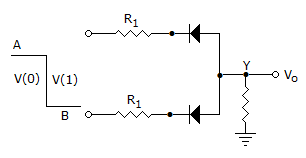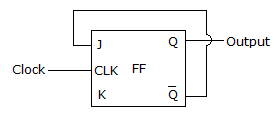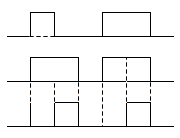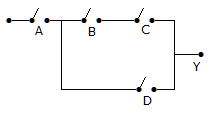# Test: Digital Electronics - 3

## 25 Questions MCQ Test Digital Electronics | Test: Digital Electronics - 3

Description
This mock test of Test: Digital Electronics - 3 for Electrical Engineering (EE) helps you for every Electrical Engineering (EE) entrance exam. This contains 25 Multiple Choice Questions for Electrical Engineering (EE) Test: Digital Electronics - 3 (mcq) to study with solutions a complete question bank. The solved questions answers in this Test: Digital Electronics - 3 quiz give you a good mix of easy questions and tough questions. Electrical Engineering (EE) students definitely take this Test: Digital Electronics - 3 exercise for a better result in the exam. You can find other Test: Digital Electronics - 3 extra questions, long questions & short questions for Electrical Engineering (EE) on EduRev as well by searching above.
QUESTION: 1

Solution:
QUESTION: 2

Solution:
QUESTION: 3

### In 8085 microprocessor, which of the following interrupts has the highest priority?

Solution:
QUESTION: 4

Schottky clamping is resorted to in TTL gates

Solution:
QUESTION: 5

The circuit shown in the figure is aSolution:
QUESTION: 6

Assertion (A): An SR latch has the problem of RAC condition

Reason (R): While designing a digital circuit RAC condition should be avoided.

Solution:
QUESTION: 7

In a clocked NAND latch, race condition occur when

Solution:
QUESTION: 8

In 1-to-4 demultiplexer, how many select lines are required?

Solution:

The formula for total no. of outputs is given by: 2^n, where n is the no. of select lines.

QUESTION: 9

In Von-Neumann-or Princeton-type computers, the program

Solution:
QUESTION: 10

Solution:
QUESTION: 11

A full adder has two outputs SUM and CARRY.

Solution:
QUESTION: 12

For the circuit shown in the figure, what is the frequency of the output Q?Solution:
QUESTION: 13

Which two rows represent identical binary numbers?

Solution:
QUESTION: 14

How many input-output ports can be accessed by direct method of 8085 μp?

Solution:
QUESTION: 15

The binary equivalent of 4096.90625 is

Solution:
QUESTION: 16

When two 16-input multiplexers drive a 2-input MUX, what is the result?

Solution:
QUESTION: 17

In floating point number 0110100000010101, the exponent is equal to __________ 10

Solution:
QUESTION: 18

Two voltages are -5 V and -10 V. In positive logic

Solution:
QUESTION: 19

figure shows three pulse train inputs to a 3-input OR gate. Assuming positive logic, the output pulse rate train in figure (b) would beSolution:
QUESTION: 20

The advantage of using dual slope ADC in digital voltmeter is that

Solution:
QUESTION: 21

Which multivibrator can be used as a clock timer?

Solution:
QUESTION: 22

T-flip-flop is commonly used as

Solution:
QUESTION: 23

In the NMOS inverter

Solution:
QUESTION: 24

For the switch circuit, taking open as 0 and closed as 1, the expression for the circuit is Y. Y is given bySolution:
QUESTION: 25

Assuming accumulator contain A 64 and the carry is set (1).What will register A and (CY) contain after RAR?

Solution: